# What is the Quadratic Formula? - Definition, Equation & Proof

An error occurred trying to load this video.

Try refreshing the page, or contact customer support.

Coming up next: Terminology of Polynomial Functions

### You're on a roll. Keep up the good work!

Replay
Your next lesson will play in 10 seconds
• 0:05 Quadratic Equations
• 0:46 History of the…
• 1:54 Solving the Quadratic Formula
• 2:40 Example
• 4:32 Proof of the Quadratic Formula
• 7:52 Lesson Summary

Want to watch this again later?

Timeline
Autoplay
Autoplay
Speed

#### Recommended Lessons and Courses for You

Lesson Transcript
Instructor: Jennifer Beddoe
The quadratic formula is a method that is used to find the roots of a quadratic equation. In this lesson, you will learn about the history of the quadratic formula, how to use it, and prove it. A quiz at the end will solidify what you've learned.

A quadratic equation is a polynomial in which the first term is of the second degree. This means that it is squared. The standard form of the quadratic equation is: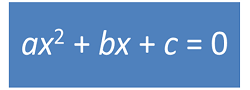There are different ways to find the roots, or solutions, of a quadratic equation. There are two solutions to every quadratic equation, however, they may or may not be real numbers. One way to find the solutions to this type of equation is by factoring. This is not always the best way to solve a quadratic equation, especially if the solutions are not whole numbers.

## The Quadratic Formula

One simple way to solve a quadratic equation is by using the quadratic formula. With just some simple math, you will have the solution quickly, whether the solution is a whole number, an irrational number, or even an imaginary number. The quadratic formula is: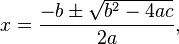## History of the Quadratic Formula

Babylonian mathematicians used a simple version of this formula as far back as 2000 B.C. The equation most closely related to the form we know today was first written down by a Hindu mathematician named Brahmagupta. Other slightly different forms followed in India and Persia.

European mathematics gained resurgence during the 1500s, and in 1545, Girolamo Cardano compiled all that was previously known about the quadratic formula and also allowed for the possibility of imaginary solutions. René Descartes, the Father of Modern Mathematics, published the quadratic formula as we know it today in his book La Géométrie.

## Solving the Quadratic Formula

If you look again at the quadratic formula, you will see that there is a symbol that looks like a plus sign over a minus sign.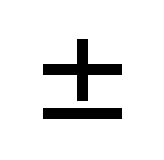This plus/minus sign means that there are really two equations to be solved, one with addition and one with subtraction.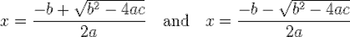It's written with the plus/minus sign to save space. To solve a quadratic equation using the quadratic formula, all you need to do is plug the proper numbers into their respective places and solve the two equations.The number in front of x squared is a, the number in front of the x is b and the number by itself with no variable is c. If there is no number in front of either x squared or x, then use the number 1. If there is no x term or no number without a variable, use 0.

## Example

Let's solve the equation y = x^2 + 2x - 3

For this problem,

a = 1
b = 2
c = -3

If you plug all of the numbers into the formula, you will get: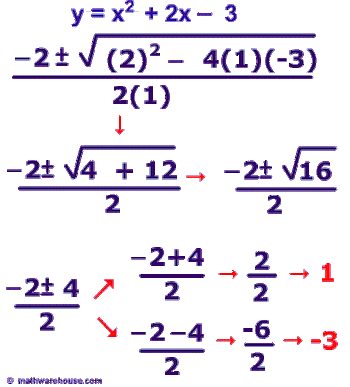Now let's figure for the two equations indicated by the plus/minus sign.• The first will be -2 plus 4 over 2, or 2 over 2, which equals 1!
• The second is -2 minus 4, or -6 over 2, which equals -3!

Now look at this example - the solutions are not as easy; however, they are much easier than trying to solve by factoring the quadratic: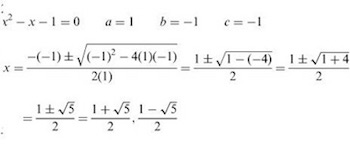Okay, so now we have x equals 1 plus or minus the square root of 5, over 2. The two equations indicated by the plus/minus sign are

• 1 plus the square root of 5, over 2
• 1 minus the square root of 5, over 2

To unlock this lesson you must be a Study.com Member.

### Register to view this lesson

Are you a student or a teacher?

### Unlock Your Education

#### See for yourself why 30 million people use Study.com

##### Become a Study.com member and start learning now.
Back
What teachers are saying about Study.com

### Earning College Credit

Did you know… We have over 160 college courses that prepare you to earn credit by exam that is accepted by over 1,500 colleges and universities. You can test out of the first two years of college and save thousands off your degree. Anyone can earn credit-by-exam regardless of age or education level.